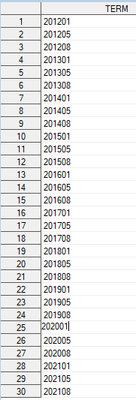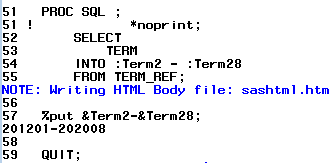## SAS Macro select into function

I am using macro function to select certain terms to use in the following programs, and use "%put" function to check whether I have selected correct terms. All I want to select is 201205 (i.e., 2nd row under TERM column) through 202101 (i.e., 28th row under TERM column). However, the output shows that terms selected are 201201-202008. How should I change the program to make correct selection? Here I attached a screen shot of the table I used. Thanks for any feedback!``````PROC SQL ; *noprint;
SELECT
TERM
INTO :Term2 - :Term28
FROM TERM_REF;

%put &Term2-&Term28;

QUIT;``````1 ACCEPTED SOLUTION

Accepted Solutions

## Re: SAS Macro select into function

If you prefer SQL, try this:

``````PROC SQL ; *noprint;
SELECT
TERM
INTO :Term2 - :Term28
FROM TERM_REF
WHERE 201205 <= Term <= 202101
;

%put &Term2-&Term28;

QUIT;``````

Jim

5 REPLIES 5

## Re: SAS Macro select into function

You can use _n_ to track the row numbers first. And then use proc sql.

``````data have;
do term=201201 to 201230;
output;
end;
run;

data keep1;
set have;
row=_n_;
run;

proc sql noprint;
select term into: term2 from keep1 where row=2;
select term into: term28 from keep1 where row=28;
quit;

%put &term2;
%put &term28;

``````

If you have more rows then you can automate it using macros. But assuming you need only 2 rows, this is the simplest way.

## Re: SAS Macro select into function

Do you always want to skip the first observation?  Then just exclude it from the input to your SELECT statement.

``FROM TERM_REF(firstobs=2)``

Or do you want to limit by the values you mentioned instead?  Are those dates with format that only displays 6 digits?  Or are the numbers like 201,201?

``where term_ref >= 201205``

## Re: SAS Macro select into function

If you prefer SQL, try this:

``````PROC SQL ; *noprint;
SELECT
TERM
INTO :Term2 - :Term28
FROM TERM_REF
WHERE 201205 <= Term <= 202101
;

%put &Term2-&Term28;

QUIT;``````

Jim

Thanks!

## Re: SAS Macro select into function

You're welcome.

Incidentally, the code could be made more flexible like this:

``````%LET	Start			=	201205;
%LET	Stop			=	202101;

PROC SQL ; *noprint;
SELECT
COUNT(TERM)	AS	Terms_Retreived
INTO	:Terms_Retrieved	TRIMMED
FROM TERM_REF
WHERE &Start <= Term <= &Stop
;
SELECT
TERM
INTO :Term1 -
FROM TERM_REF
WHERE &Start <= Term <= &Stop
;
QUIT;

%IF	%BQUOTE(&Terms_Retrieved)	%THEN
%DO;
%put	NOTE:  &Term1-&&Term&Terms_Retrieved;
%END;``````

With the above code, you don't need to know in advance how many terms (or which terms) will be retrieved.  SAS will figure out how many terms are in the range you specify via the %LET statements, create the appropriate number of macro variables, and display the range in the log for you.

Jim

Discussion stats
• 5 replies
• 593 views
• 2 likes
• 4 in conversation